# The Instant Insanity Game.ppt

29 de Mar de 2023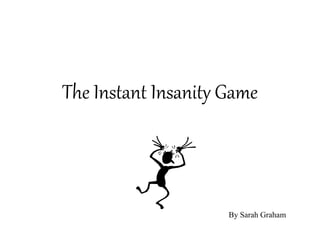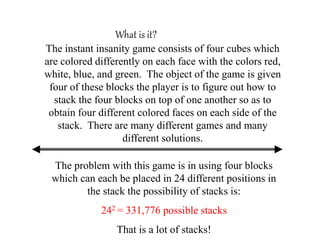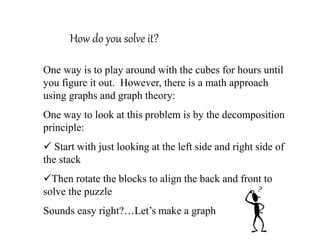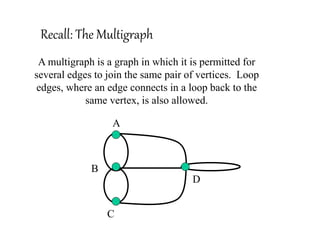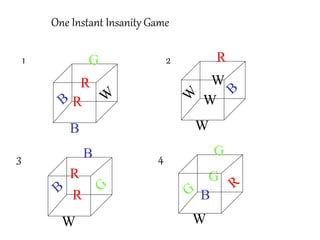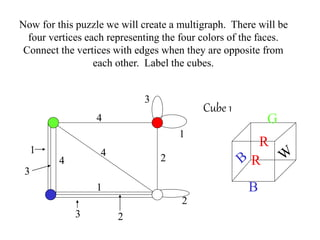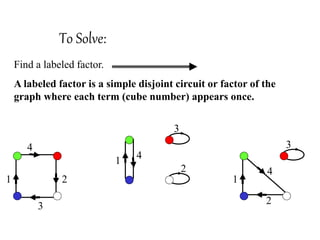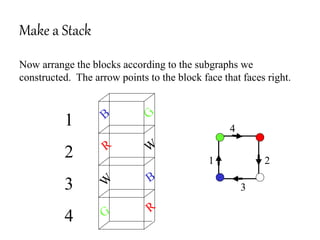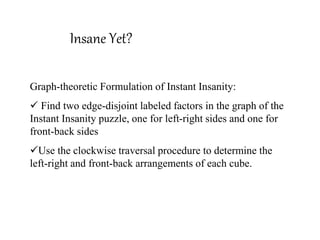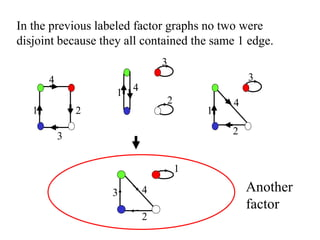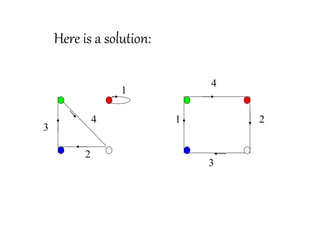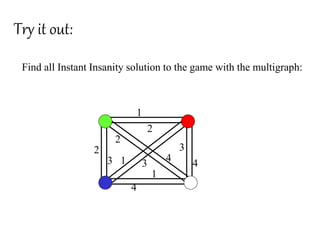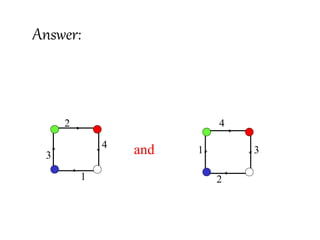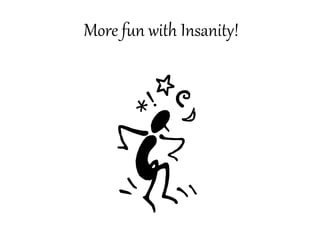1 de 14

### The Instant Insanity Game.ppt

1. The Instant Insanity Game By Sarah Graham
2. What is it? The instant insanity game consists of four cubes which are colored differently on each face with the colors red, white, blue, and green. The object of the game is given four of these blocks the player is to figure out how to stack the four blocks on top of one another so as to obtain four different colored faces on each side of the stack. There are many different games and many different solutions. The problem with this game is in using four blocks which can each be placed in 24 different positions in the stack the possibility of stacks is: 242 = 331,776 possible stacks That is a lot of stacks!
3. How do you solve it? One way is to play around with the cubes for hours until you figure it out. However, there is a math approach using graphs and graph theory: One way to look at this problem is by the decomposition principle:  Start with just looking at the left side and right side of the stack Then rotate the blocks to align the back and front to solve the puzzle Sounds easy right?…Let’s make a graph
4. Recall: The Multigraph A multigraph is a graph in which it is permitted for several edges to join the same pair of vertices. Loop edges, where an edge connects in a loop back to the same vertex, is also allowed. A B C D
5. One Instant Insanity Game R G B W W W W W R R R G B R 1 2 3 4
6. Now for this puzzle we will create a multigraph. There will be four vertices each representing the four colors of the faces. Connect the vertices with edges when they are opposite from each other. Label the cubes. 1 1 1 2 2 2 3 3 3 4 4 4 R G B R Cube 1
7. To Solve: Find a labeled factor. A labeled factor is a simple disjoint circuit or factor of the graph where each term (cube number) appears once. 1 2 3 4 1 2 3 4 1 2 3 4
8. Make a Stack Now arrange the blocks according to the subgraphs we constructed. The arrow points to the block face that faces right. 1 2 3 4 1 2 3 4
9. Insane Yet? Graph-theoretic Formulation of Instant Insanity:  Find two edge-disjoint labeled factors in the graph of the Instant Insanity puzzle, one for left-right sides and one for front-back sides Use the clockwise traversal procedure to determine the left-right and front-back arrangements of each cube.
10. In the previous labeled factor graphs no two were disjoint because they all contained the same 1 edge. 1 2 3 4 1 2 3 4 1 2 3 4 1 2 3 4 Another factor
11. 1 2 3 4 1 2 4 Here is a solution: 3
12. Try it out: Find all Instant Insanity solution to the game with the multigraph: 1 2 3 4 1 1 2 2 3 3 4 4
13. Answer: 1 2 3 4 1 2 3 4 and
14. More fun with Insanity!